FanDuel World Cup Winner Odds
+450
4.5 to 1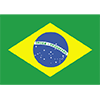Brazil
15.7% implied probability

+550
5.5 to 1France
13.3% implied probability

No changes have been recorded yet.
+700
7 to 1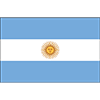Argentina
10.8% implied probability

+750
7.5 to 1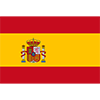Spain
10.2% implied probability

+750
7.5 to 1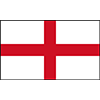England
10.2% implied probability

+950
9.5 to 1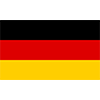Germany
8.2% implied probability

No changes have been recorded yet.
+1300
13 to 1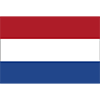Netherlands
6.2% implied probability

No changes have been recorded yet.
+1700
17 to 1Belgium
4.8% implied probability

+1700
17 to 1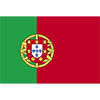Portugal
4.8% implied probability

+3200
32 to 1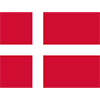Denmark
2.6% implied probability

No changes have been recorded yet.
+4900
49 to 1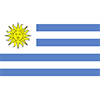Uruguay
1.7% implied probability

+4900
49 to 1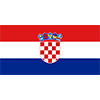Croatia
1.7% implied probability

+7500
75 to 1Switzerland
1.1% implied probability

+9000
90 to 1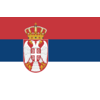Serbia
0.9% implied probability

+9000
90 to 1Senegal
0.9% implied probability

+9000
90 to 1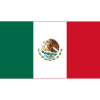Mexico
0.9% implied probability

+12000
120 to 1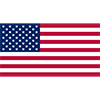USA
0.7% implied probability

+12000
120 to 1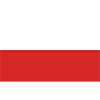Poland
0.7% implied probability

+12000
120 to 1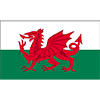Wales
0.7% implied probability

+15000
150 to 1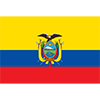Ecuador
0.6% implied probability

+21000
210 to 1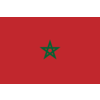Morocco
0.4% implied probability

+21000
210 to 1Cameroon
0.4% implied probability

No changes have been recorded yet.
+25000
250 to 1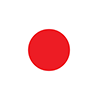Japan
0.3% implied probability

+25000
250 to 1Ghana
0.3% implied probability

+25000
250 to 1South Korea
0.3% implied probability

+25000
250 to 1Canada
0.3% implied probability

+55000
550 to 1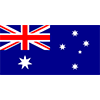Australia
0.2% implied probability

+55000
550 to 1Iran
0.2% implied probability

No changes have been recorded yet.
+55000
550 to 1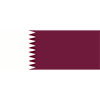Qatar
0.2% implied probability

+55000
550 to 1Saudi Arabia
0.2% implied probability

No changes have been recorded yet.
+55000
550 to 1Costa Rica
0.2% implied probability

No changes have been recorded yet.
+55000
550 to 1Tunisia
0.2% implied probability